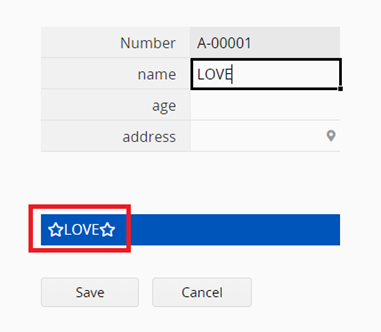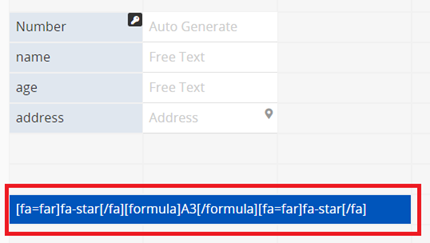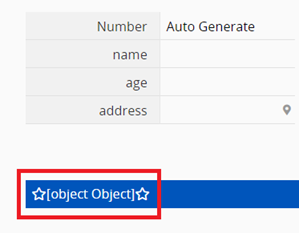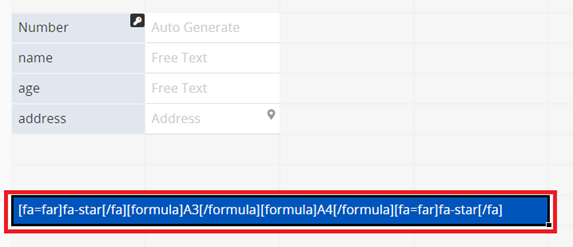Cant apply two BB code in a single description

#1

Hi ragic members,
I testing on the bb code.
however, i trying to apply my bb code twice inside a description.
but i cannot show…
the bb code that i using is [formula]A31[/formula]

bb code like this [fa=far]fa-star[/fa] is working well

when i apply two [formula]A3[/formula] bb code, it turns into Object Object??
What does it mean?

How to apply two [formula]A3[/formula] bb code in a single description?

Thank you

Photo for your reference~#2

Hi,

[formula]A3+A4[/formula]

#3

if in between this A3 and A4, i would like to add text in between.
how to do it?

thanks.

#4

Hi Angie~
I got it~
Thanks